Courses

# Bessel's Special Function (Part - 5) - Mathematical Methods of Physics, UGC - NET Physics Physics Notes | EduRev

## Physics for IIT JAM, UGC - NET, CSIR NET

Created by: Akhilesh Thakur

## Physics : Bessel's Special Function (Part - 5) - Mathematical Methods of Physics, UGC - NET Physics Physics Notes | EduRev

The document Bessel's Special Function (Part - 5) - Mathematical Methods of Physics, UGC - NET Physics Physics Notes | EduRev is a part of the Physics Course Physics for IIT JAM, UGC - NET, CSIR NET.
All you need of Physics at this link: Physics

SPHERICAL BESSEL FUNCTIONS

When the Helmholtz equation is separated in spherical coordinates, the radial equation has the form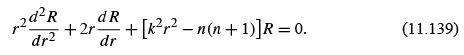This is Eq. (9.65) of Section 9.3. The parameter k enters from the original Helmholtz equation, while n(n + 1) is a separation constant. From the behavior of the polar angle function (Legendre’s equation, Sections 9.5 and 12.5), the separation constant must have this form, with n a nonnegative integer. Equation (11.139) has the virtue of being selfadjoint, but clearly it is not Bessel’s equation. However, if we substitute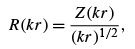Equation (11.139) becomes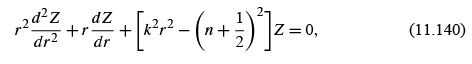which is Bessel’s equation. Z is a Bessel function of order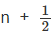(n an integer). Because of the importance of spherical coordinates, this combination, that is,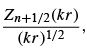occurs quite often.

Deﬁnitions

It is convenient to label these functions spherical Bessel functions with the following deﬁning equations: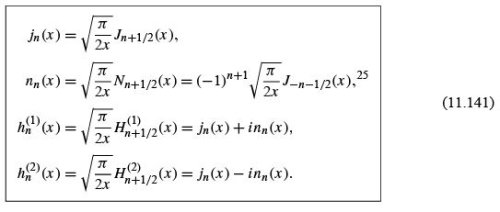These spherical Bessel functions (Figs. 11.13 and 11.14) can be expressed in series form by using the series (Eq. (11.5)) for Jn , replacing n with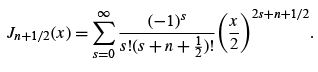(11.142)

Using the Legendre duplication formula,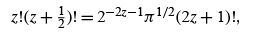(11.143)

we have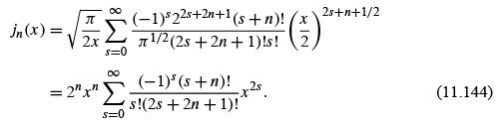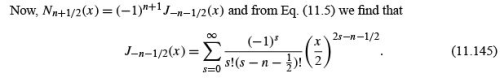This yields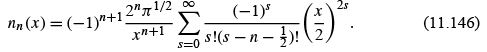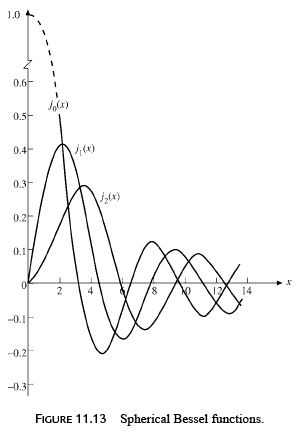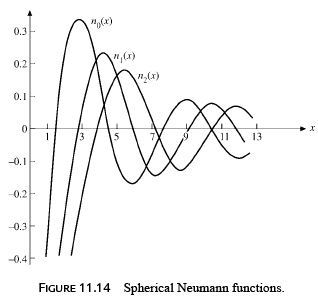The Legendre duplication formula can be used again to give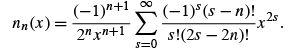(11.147)

These series forms, Eqs. (11.144) and (11.147), are useful in three ways: (1) limiting values as x → 0, (2) closed-form representations for n = 0, and, as an extension of this, (3) an indication that the spherical Bessel functions are closely related to sine and cosine.
For the special case n = 0 we ﬁnd from Eq. (11.144) that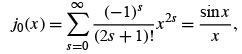(11.148)

whereas for n0 , Eq. (11.147) yields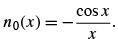(11.149)

From the deﬁnition of the spherical Hankel functions (Eq. (11.141)),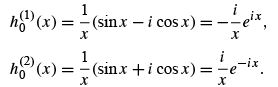(11.150)

Equations (11.148) and (11.149) suggest expressing all spherical Bessel functions as combinations of sine and cosine. The appropriate combinations can be developed from the power-series solutions, Eqs. (11.144) and (11.147), but this approach is awkward. Actually the trigonometric forms are already available as the asymptotic expansion of Section 11.6.
From Eqs. (11.131) and (11.129a),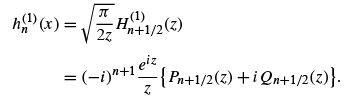(11.151)

Now, Pn+1/2   and Qn+1/2 are polynomials. This means that Eq. (11.151) is mathematically exact, not simply an asymptotic approximation. We obtain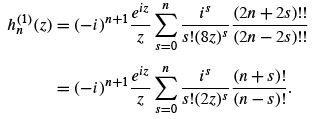(11.152)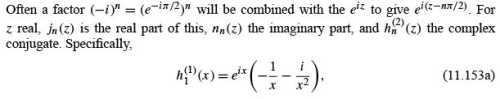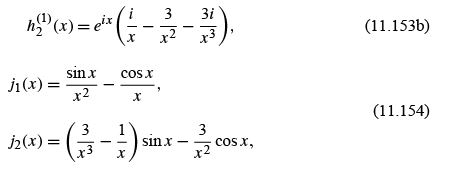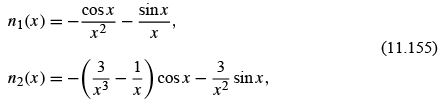and so on.

Limiting Values

For x ≪ 1,26 Eqs. (11.144) and (11.147) yield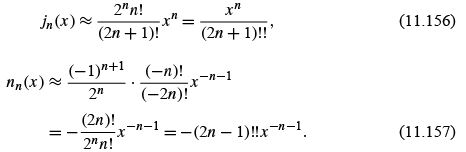The transformation of factorials in the expressions for nn (x ) employs Exercise 8.1.3. The limiting values of the spherical Hankel functions go as ±inn (x ).
The asymptotic values of jn ,nn ,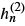, and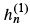may be obtained from the Bessel asymptotic forms, Section 11.6. We ﬁnd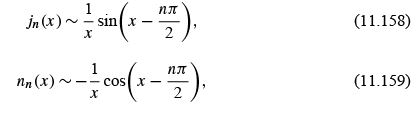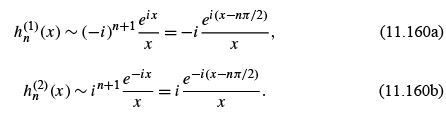The condition for these spherical Bessel forms is that x ≫ n(n + 1)/2. From these asymptotic values we see that jn (x ) and nn (x ) are appropriate for a description of standing spherical waves;(x ) and(x ) correspond to traveling spherical waves. If the time dependence for the traveling waves is taken to be e−iωt , then(x ) yields an outgoing traveling spherical wave,(x ) an incoming wave. Radiation theory in electromagnetism and scattering theory in quantum mechanics provide many applications.

Recurrence Relations

The recurrence relations to which we now turn provide a convenient way of developing the higher-order spherical Bessel functions. These recurrence relations may be derived from the series, but, as with the modiﬁed Bessel functions, it is easier to substitute into the known recurrence relations (Eqs. (11.10) and (11.12)). This gives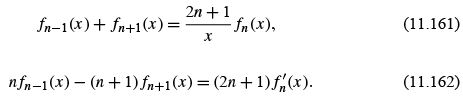Rearranging these relations (or substituting into Eqs. (11.15) and (11.17)), we obtain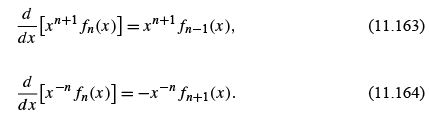Here fmay represent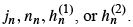The speciﬁc forms, Eqs. (11.154) and (11.155), may also be readily obtained from Eq. (11.164).
By mathematical induction we may establish the Rayleigh formulas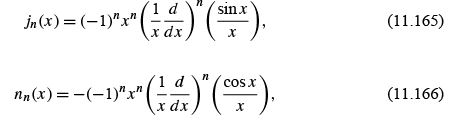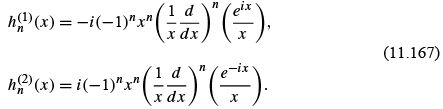Orthogonality

We may take the orthogonality integral for the ordinary Bessel functions (Eqs. (11.49) and (11.50)),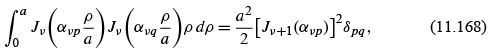and substitute in the expression for jn to obtain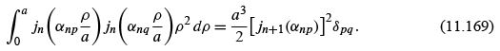Here αnp and αnq are roots of jn .
This represents orthogonality with respect to the roots of the Bessel functions. An illustration of this sort of orthogonality is provided in Example 11.7.1, the problem of a particle in a sphere. Equation (11.169) guarantees orthogonality of the wave functions jn (r ) for ﬁxed n. (If n varies, the accompanying spherical harmonic will provide orthogonality.)

Example 11.7.1 PARTICLE IN A SPHERE

An illustration of the use of the spherical Bessel functions is provided by the problem of a quantum mechanical particle in a sphere of radius a . Quantum theory requires that the wave function ψ , describing our particle, satisfy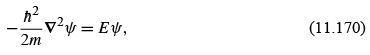and the boundary conditions (1) ψ(r ≤ a) remains ﬁnite, (2) ψ(a ) = 0. This corresponds to a square-well potential V = 0, r ≤ a , and V =∞, r> a .Here h¯ is Planck’s constant divided by 2π, m is the mass of our particle, and E is, its energy. Let us determine the minimum value of the energy for which our wave equation has an acceptable solution.
Equation (11.170) is Helmholtz’s equation with a radial part (compare Section 9.3 for separation of variables):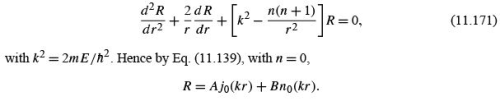We choose the orbital angular momentum index n = 0, for any angular dependence would raise the energy. The spherical Neumann function is rejected because of its divergent behavior at the origin. To satisfy the second boundary condition (for all angles), we require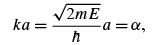(11.172)

where α is a root of j, that is, j0 (α) = 0. This has the effect of limiting the allowable energies to a certain discrete set, or, in other words, application of boundary condition (2) quantizes the energy E . The smallest α is the ﬁrst zero of j0 ,

α = π,

and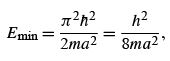(11.173)

which means that for any ﬁnite sphere the particle energy will have a positive minimum or zero-point energy. This is an illustration of the Heisenberg uncertainty principle for Δp with Δr ≤ a .
In solid-state physics, astrophysics, and other areas of physics, we may wish to know how many different solutions (energy states) correspond to energies less than or equal to some ﬁxed energy E0 . For a cubic volume (Exercise 9.3.5) the problem is fairly simple.
The considerably more difﬁcult spherical case is worked out by R. H. Lambert, Am. J.
Phys. 36: 417, 1169 (1968).
The relevant orthogonality relation for the jn (kr ) can be derived from the integral given in Exercise 11.7.23.

Another form, orthogonality with respect to the indices, may be written as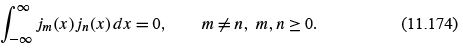The proof is left as Exercise 11.7.10. If m = n (compare Exercise 11.7.11), we have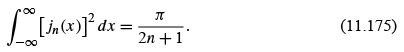Most physical applications of orthogonal Bessel and spherical Bessel functions involve orthogonality with varying roots and an interval [0,a ] and Eqs. (11.168) and (11.169) and Exercise 11.7.23 for continuous-energy eigenvalues.
The spherical Bessel functions will enter again in connection with spherical waves, but further consideration is postponed until the corresponding angular functions, the Legendre functions, have been introduced.

159 docs

,

,

,

,

,

,

,

,

,

,

,

,

,

,

,

,

,

,

,

,

,

,

,

,

;